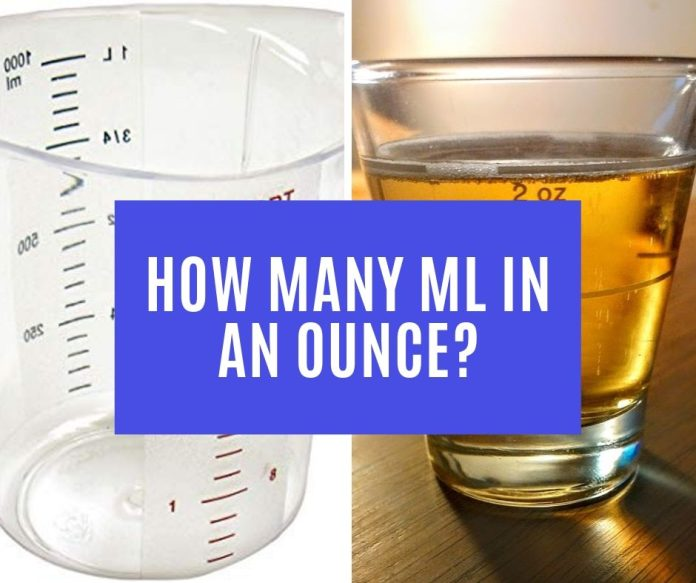# How Many mL in an Ounce?

0
2862mL and ounce are units of measurement commonly used to measure volume capacity. Supermarket labels for liquid ingredients use mL while actual beverages in bottles usually use ounce.

mL is not that complex but ounce is because it is divided between the dry ounce and the fluid ounce. For this matter, we need to clarify that the ounce that we will be talking about here is the fluid ounce. mL to ounce is a useful conversion most specifically for liquid ingredients. So how many mL is in an ounce?

[CP_CALCULATED_FIELDS id=”32″]

## What is mL?

Milliliters which when abbreviated is mL is a unit of measurement which accounts for volume capacity. It is mostly used to measure small amounts of liquid ingredients.

According to Math is Fun, one liter is equal to 1,000 mL. One mL is also equivalent to 20 drops. mL is used every day activities such as in measuring liquid medicine and liquid ingredients for cooking.

1 liter = 1,000 mL

1 mL = 20 drops

## How many mL in an Ounce?

This depends on which part of the world you are in but the standard conversion value is lifted from the US metric system which is 29.57 mL is to one fluid ounce, according to The Calculator Site. The same conversion value is used by Canada.

1 US fluid ounce = 29.57 mL

However, aside from this conversion value, there is also the imperial/UK fluid ounce whose conversion value is 28.41.

1 UK/imperial fluid ounce = 28.41 mL

But in actuality, these values may somehow be inexact. For example, the average 4 ounces of ice cream is calculated at 120mL and not 118.28. The same is true for cooking oil. But exact conversions can be found in substances like chemicals for experiments and in medicines.

4 ounces of ice cream = 120 mL

## How to convert ounces to mL?

[CP_CALCULATED_FIELDS id=”32″]

So how do we convert ounces to mL? All we have to do is to multiply 29.57 with the value of ounces that we need to find out the mL conversion of. We use 29.57 because it is considered as the standard conversion value for mL to ounce.

n ounces = 29.57 x n mL

n: number of ounces

## Ounces to mL conversion table

Since we have stated that there is also an imperial/UK fluid ounce, we provide the table below for ease of conversion and also for comparison.

1.7 oz to mL = 50.269 mL
2 oz to mL = 59.14 mL
3.4 oz to mL = 100.538 mL
4 oz to mL = 118.28 mL
6 oz to ml = 177.42 mL
10 oz to ml = 295.7 mL
21 oz to ml = 620.97 mL
40 oz to ml = 1182.8 mL

 US Fluid Ounce mL UK/Imperial Fluid Ounce mL 1 29.57 1 28.41 2 59.14 2 56.82 3 88.71 3 85.23 4 118.28 4 113.64 5 177.55 5 142.05

It is really not that hard. All you need to remember is the base conversion value of mL to ounce which is 29.57. This may also come in handy when you are not in the US for example since recipe books would specifically indicate the metric system needed for conversion.

## Conclusion

We have already summarized mL to ounces conversion and we mentioned that the standard conversion value used for mL to ounce is 29.57. However, it must also be noted that there exists an imperial/UK conversion value too which is 28.41.

Nevertheless, it is really beneficial for us to know the mL to ounce conversion because these two units of measurement are very common in measuring liquid products specifically beverages and liquid ingredients.